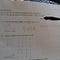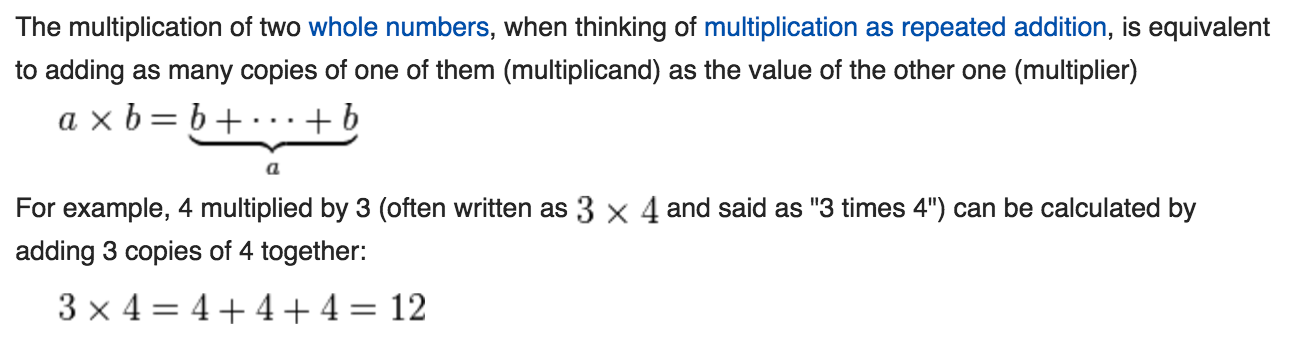# Why Was 5 x 3 = 5 + 5 + 5 Marked Wrong

## Viral Math Problem Explained

A lot of you have been asking about WHY this viral math problem is marked incorrect. And that’s a great question! It seems a little absurd, doesn’t it?Cloakenn/Imgur

# Equals Versus Equivalency

Just because two things are equal doesn’t mean they’re equivalent.

Equal is defined as, “being the same in quantity, size, degree, or value.” Whereas equivalent is defined as, “equal in value, amount, function, or meaning.” In the above problem 5 x 3 is equal to 5 + 5 + 5, but they’re not necessarily equivalent. Equivalence relates to meaning, so it depends on the meaning of multiplication.

# I did something today I’ve never done before,

## I looked up the definition of multiplication.

To be able to apply the commutative property, we must first establish a definition of multiplication that applies meaning to the operand order. If operand order isn’t important, the commutative property serves no useful distinction.

In Wikipedia’s definition of multiplication, they indicate the first factor is the number of copies and the second is the number being repeated.“Multiplication”, Wikipedia

If this is the definition the teacher taught, 5 x 3 is equivalent to 5 copies of 3, or 3 + 3 + 3 + 3 + 3. It is equal but not equivalent to 5 + 5 + 5 because 3 copies of 5 represents something different.

For example, 3 bundles of 5 bananas is different from 5 bundles of 3 bananas although they total to the same number of bananas. Their structures are different.

Here’s another example: 30 ÷ 2 is equal to 15. But does 30 ÷ 2 represent multiplication? Is it equivalent to repeated addition?

No, it represents division. It is equal to 5 times 3 but not equivalent.

# I get the difference, but isn’t it a little harsh?

It depends. If the teacher has already taught the commutative property of multiplication (the law that says a x b = b x a), then this is a fine substitution to make. And it is awesome that the student realized this! Kudos! What a mathlete!

If the teacher has not covered the commutative property, then it might be unwise to let a student continue with this line of thought if they don’t understand the reasons why fully.

It’s common for beginners to get confused as to when it is okay to switch the order of values in binary operations. We know that the following are not equal.

But this is easily confused with the child who sees that sometimes it’s okay to switch the order and other times it’s not and hasn’t learned when or why.

By focusing on the meaning of these operations as they relate to repeated addition, arrays and area, teachers are creating a deeper understanding and trying to prevent students from making these kinds of mistakes.

# But It’s the Right Answer,

## Why does it matter if the meanings are slightly different?

It’s more important than ever for students to understand the difference between equal as a result and equivalence in meaning from a young age because it is a fundamental computer science concept.

In programming, there is a distinction between testing if two things are equal or equivalent (aka identical).

Equal means they have the same end value, like 5 + 5 + 5 = 3 • 5 = 5 • 3 = 15. Equivalent means not only are they equal, they are also of the same data type. In other words, they mean the same thing.

Depending on the language, numbers and expressions that look the same don’t always mean the same.

For example, in JavaScript if we test for equality with the == operator:

• “4” == 4 returns True

because the compiler understands both are referring to the number 4. But if we test for identity using the === operator:

• “4” === 4 returns False

because they mean different things. The first is a string whereas the second is a number, therefore they are not the same. This is just one example of how equality isn’t always straightforward.

(Note: for a more in depth discussion on == vs === in JS, check out this stack overflow discussion.)

# The Right Mindset for Matrix Multiplication

Notice that the second problem is marked incorrect as well. Why is it important that 4 x 6 is 4 rows of 6, instead of 6 rows of 4?

Not only does this adhere to the definition, it also teaches students the correct order for diagramming matrices, which is rows times columns.

Keeping rows and columns straight in matrix multiplication is vital.

Matrices are labeled using a row by column notation, m x n. To multiply matrices together, you multiply the rows of the first matrix by the columns of the second. The number of columns in the first matrix must equal the number of rows in the second, or else you cannot multiply them together.

For example, we can multiply a 2 x 3 matrix and a 3 x 4 matrix together. But if we swap the order there will not be sufficient rows and columns, and the operation cannot be performed.

Order is essential in the definition of multiplication because not all forms of multiplication are commutative, such as matrix multiplication. This is why it is taught as a separate property.

# Respect the Teachers

They are qualified experts on child education. They have the best intentions for the students in mind. This teacher made a decision based off a lot more information about the student and class setting than we can tell from a photo. We don’t have to agree with it, but we can respect it. If you are confused, ask them why they did something before you discredit a teacher on the internet.

## Math Hacks

Tutorials with a fresh perspective.

Written by

## Brett Berry

Check out my YouTube channel “Math Hacks” for hands-on math tutorials and lots of math love ♥️## Math Hacks

Tutorials with a fresh perspective.

## More From Medium

Medium is an open platform where 170 million readers come to find insightful and dynamic thinking. Here, expert and undiscovered voices alike dive into the heart of any topic and bring new ideas to the surface. Learn more

Follow the writers, publications, and topics that matter to you, and you’ll see them on your homepage and in your inbox. Explore

If you have a story to tell, knowledge to share, or a perspective to offer — welcome home. It’s easy and free to post your thinking on any topic. Write on Medium